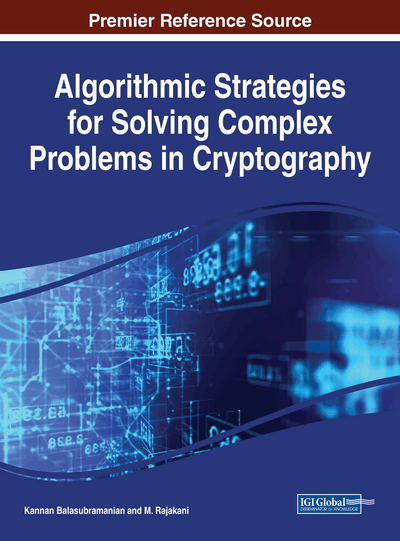# The Quadratic Sieve Algorithm for Integer Factoring

Kannan Balasubramanian (Mepco Schlenk Engineering College, India) and M. Rajakani (Mepco Schlenk Engineering College, India)
DOI: 10.4018/978-1-5225-2915-6.ch018
Available
\$37.50
No Current Special Offers

## Abstract

At the time when RSA was invented in 1977, factoring integers with as few as 80 decimal digits was intractable. The first major breakthrough was quadratic sieve, a relatively simple factoring algorithm invented by Carl Pomerance in 1981, which can factor numbers up to 100 digits and more. It's still the best-known method for numbers under 110 digits or so; for larger numbers, the general number field sieve (GNFS) is now used. However, the general number field sieve is extremely complicated, for even the most basic implementation. However, GNFS is based on the same fundamental ideas as quadratic sieve. The fundamentals of the Quadratic Sieve algorithm are discussed in this chapter.
Chapter Preview
Top

## Finding A Subset Of Integers Whose Product Is A Square

Suppose I give you a set of integers and I ask you to find a subset of those integers whose product is a square, if one exists. For example, given the set {10, 24, 35, 52, 54, 78}, the product 24×52×78 is 97344 = 3122. The brute-force solution, trying every subset, is too expensive because there are an exponential number of subsets.

Another approach is based on prime factorizations and linear algebra. First, we factor each of the input numbers into prime factors; for now, we will assume that these numbers are easy to factor. For the above example set, we get:

10 = 2 × 524 = 23 × 335 = 5 × 752 = 22 × 1354 = 2 × 3378 = 2 × 3 × 13

When you multiply two numbers written as prime factorizations, you simply add the exponents of the primes used. For example, the exponent of 2 in 24×52×78 is 6, because it’s 3 in 24, 2 in 52, and 1 in 78. A number is a square if and only if all the exponents in its prime factorization are even. Suppose we write the above factorizations as vectors, where the kth entry corresponds to the exponent of the kth prime number. We get:

[1 0 1 0 0 0][3 1 0 0 0 0][0 0 1 1 0 0][2 0 0 0 01] [1 3 0 0 0 0][1 1 0 0 01]

Now, multiplying numbers is as simple as adding vectors. If we add rows 2, 4, and 6, we get [6 2 0 0 0 2], which has all even exponents and so must be a square. In more familiar terms, we want the last bit of each entry in the sum to be zero. But in this case, we don’t need to store all of the numbers above, only the last bit of each number. This gives us the following:

[1 0 1 0 0 0][1 1 0 0 0 0][0 0 1 1 0 0][0 0 0 0 01] [1 1 0 0 0 0][1 1 0 0 01]

Moreover, since we’re only interested in last bits, we can perform all our addition using one-bit integers with wraparound semantics (in other words, mod 2). If we add rows 2, 4, and 6 in this way, we get [0 0 0 0 0 0 0], the zero vector. In fact, all squares correspond to the zero vector.

Let’s rephrase this as a matrix equation problem. If we transpose the above matrix, so that rows become columns, we get this:

[1 1 0 0 11] [0 1 0 0 11] [1 0 1 0 0 0][0 0 1 0 0 0][0 0 0 0 0 0][0 0 0 1 01]

Call this matrix A. If we multiply A by the vector [0 1 0 1 0 1], using one-bit integer arithmetic, we get the zero vector. This tells us precisely which numbers we need to multiply to get a square. So, our goal is to find a nonzero vector x such that Ax=0 (remember, all arithmetic here is with one-bit integers).

## Complete Chapter List

Search this Book:
Reset